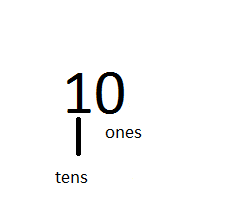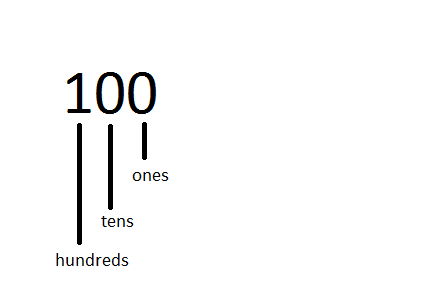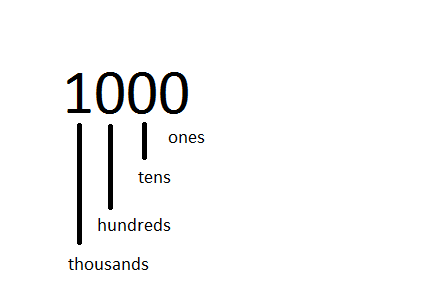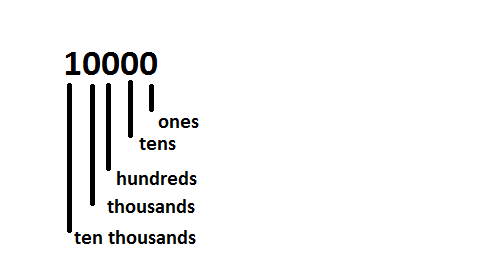Checkout JEE MAINS 2022 Question Paper Analysis : Checkout JEE MAINS 2022 Question Paper Analysis :

# Digits

Digits are the single symbols used to represent numbers in Maths. For example, in number 89, 8 and 9 are two digits. Hence, the numerals such as 0, 1, 2, 3,4, 5,6,7,8,9, and 0 are the form of digits which are used to represent a combination of numbers and do arithmetic operations in our day to day life.

The term digits are preferably used in computer science. In mathematics, these digits are said to be numerical digits or sometimes simply numbers. The smallest one-digit number is 1 and the largest one-digit number is 9.

## History of digits

Thousands of years ago, when the number system was not known to people, roman abacus or stone tokens were used. With epoch of time and advancements in trade across regions and countries, the need for bigger denominations was felt. This led to the introduction of the number systems, as we know today.  As the nations progressed, the need to deal with bigger numbers also came forth. The distance between the earth and the moon, the speed of light, the size of a microorganism; these curiosities instilled in us, the need for expanding our number systems. The concept of numbers and digits was introduced hence.

Counting small numbers is very easy. The petals of a flower, the fingers in our hand, we can count these numbers very easily, without the aid of a calculator or a pen and paper. In such cases, we deal with numbers with a single digit. The smallest single digit counting number is 1 whereas the greatest is 9.

Now imagine the students in your class. Can u count them easily? One might say yes for a small class of forty to eighty students. Similarly, for counting the buildings in a locality, the number of general stores in a locality and so on. To keep a track of such measures, we use numbers up to two digits.

### Two digits numbers

When we add one unit to the greatest one-digit number we get the smallest two-digit number.

1+9 = 10

The smallest two-digit number is 10 and the greatest is 99.

Now imagine an auditorium, filled with hundreds of people. We definitely cannot count these many people, just by using our fingertips. In order to deal with such measures, we use numbers up to three digits.### Three Digit Numbers

When we add one unit to the greatest two-digit number we get the smallest three-digit number.

1+99 = 100

The smallest three-digit number is 100 and the greatest is 999.

Now, imagine the crowd in a sports pavilion, filled with thousands of people. Or say, the cost of a cupboard or a bicycle, in order to deal with such measures we use digits up to four digits.### Four Digit Numbers

When we add one unit to the greatest three-digit number we get the smallest four-digit number.

1+999 = 1000

The smallest four-digit number is 1000 and the greatest is 9999.

When we deal with numbers as big as the population of a state, or the cost of the motorcycle, we need to deal with numbers that go on to five digits.### Five Digit Numbers

When we add one unit to the greatest four-digit number we get the smallest five-digit number.

1+9999 = 10000The smallest five-digit number is 10000 and the greatest five-digit number is 99999.